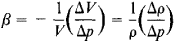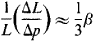# compressibility

Also found in: Dictionary, Thesaurus.
Related to Adiabatic compressibility: Isothermal compressibility

## compressibility

[kəm‚pres·ə′bil·əd·ē]
(mechanics)
The property of a substance capable of being reduced in volume by application of pressure; quantitively, the reciprocal of the bulk modulus.

## Compressibility

the ability of a substance to contract in volume under the action of hydrostatic pressure. All substances have compressibility. If a substance does not undergo, for example, chemical or structural changes in the process of compression, the original volume is restored when the external pressure returns to its initial value. In solids, which have pores, cracks, and other structural inhomogeneities, essentially reversible compressibility can be observed only at sufficiently high pressure—for example, in rocks at a pressure greater than 2–5 kilobars (1 kbar = 108newtons/m2).

The capability of reversible change in the volume V occupied by a substance under hydrostatic pressure p is usually called the elasticity of bulk, or elasticity of compression. The compressibility of the substance is characterized by the coefficient β, which is in fact called the compressibility. This coefficient expresses the relative change in the volume of a body when the pressure p is increased by one unit:where ΔV and Δρ are the changes in the volume V and density ρ when ρ changes by the amount Δρ. K = 1/β is called the bulk modulus. For solids, K = /3(3μE), where E is Young’s modulus and μ is the shear modulus. For ideal gases, K = ρ at any temperature T. In the general case, the compressibility of a substance, and consequently K and β, depend on ρ and T. As a rule, β decreases with increasing ρ and increases with T. Compressibility is often characterized by the relative density δ = ρ/ρ0, where ρ0 is the density of 0°C and ρ = 1 atmosphere (atm).

Compression can occur isothermally—that is, at a constant temperature—or with simultaneous heating of the body undergoing compression—for example, in an adiabatic process. In the latter case, the values of K will be greater than in the case of isothermal compression; the difference is a few percent for most solids at normal temperatures.

Equations of state that express the relation between p, V, and T are used over a broad range of pressures to estimate the compressibility of substances. The compressibility can be determined directly from the change in the volume of bodies under pressure (seePIEZOMETER), from acoustical measurements of the velocity of propagation of elastic waves in the substance, or from experiments on shock compression that give the relation between ρ and p at the maximum experimentally produced pressures. The compressibility can also be found from X-ray diffraction measurements of the parameters of a crystal lattice under pressure. What is known as the linear compressibility can be determined by measuring the linear deformation of a solid under hydrostatic pressure. For an isotropic body, the linear compressibility isThe compressibility of gases is very large at pressures up to 1 kbar but becomes close to that of liquids as the density of the gases approaches the density of liquids. The compressibility of liquids decreases with increasing p, at first abruptly and then very slowly. As p increases from 6 kbar to 12 kbar, β decreases by approximately the same factor as it does when p increases from 1 atm (10-3 kbar) to 1 kbar—that is, by a factor of approximately 2. At 10–12 kbar, β is 5–10 percent of its initial value. At 30–50 kbar, the K of liquids is close in order of magnitude to the K of solids. For solids at 100 kbar, Δρ/ρ0 ≈ 15–25 percent. For some substances, such as the alkali metals, Δρ/ρ ~ 40 percent, and for most other metals it is ~6–15 percent. The linear compressibility of anisotropic substances depends on the crystallographic directions (in any case, up to pressures of tens of kilo-bars), and along the directions with weak interatomic interaction it can be eight to ten times greater than the compressibility in directions along which there is stronger bonding in the crystal lattice; the change in the lattice parameter in these directions in a certain range of p can even be positive—for example, in tellurium and selenium. The compressibility is the most important characteristic of a substance; it permits assessment of the dependence of the substance’s physical properties on the interatomic or intermolecular distances.

The compressibility of gases (vapors), liquids, and solids must be known to calculate, for example, the operation of heat engines, industrial chemical processes, the action of an explosion, and aerodynamic and hydrodynamic effects observed during motion at high velocities. Examples of the compressibility of various substances are given in HIGH PRESSURE.

### REFERENCES

Tablitsy fizicheskikh velichin. Moscow, 1976.
Vargaftik, N. B. Spravochnik po teplofizicheskim svoistvam gazov i zhidkostei, 2nd ed. Moscow, 1972.
Spravochnik fizicheskikh konstant gornykh porod. Moscow, 1969. Chapter 7. (Translated from English.)
“Szhimaemost’.” In Fizicheskii entsiklopedicheskii slovar’, vol. 4. Moscow, 1965.

L. D. LIVSHITS

## compressibility

The relative resistance (e.g., of a soil mass) to a change in volume upon being subjected to a compressive stress.

## compressibility

A general term covering the effects resulting from the fact that the density of air, and gases in general, varies with pressure and hence with wind speed. The effects become apparent in the changes in the values of lift and drag coefficients of a wing or an airfoil above the Mach number = 0.4 and are marked as the speed of sound is approached or exceeded. The general meaning of compressibility is the ability of the material to be reduced in volume by the application of pressure.
References in periodicals archive ?
Caption: Figure 4: Variation of adiabatic compressibility with concentration at 35[degrees] C temperature.
Concentration (m/v) Ultrasonic velocity at 35[degrees] C 1.0% 1509.9 0.8% 1503.2 0.6% 1501.3 0.5% 1495.9 0.4% 1483.8 0.3% 1482.5 Table 4: Adiabatic compressibility (x [10.sup.-10] [kg.sup.-1] [ms.sup.2]) at different concentration at 1 MHz for polyvinyl alcohol (PVA).